## diffraction structural biologyJOURNAL OFSYNCHROTRONRADIATION
ISSN: 1600-5775
Volume 15| Part 3| May 2008| Pages 269-272

## Protein crystallization in a 100 nl solution with new stirring equipment

aGraduate School of Engineering, Osaka University, Suita, Osaka 565-0871, Japan, bCREST JST, Suita, Osaka 565-0871, Japan, cSOSHO Inc., Osaka 541-0053, Japan, and dInstitute of Scientific and Industrial Research, Osaka University, Ibaraki, Osaka 567-004, Japan
*Correspondence e-mail: mori.yusuke@eei.eng.osaka-u.ac.jp

(Received 1 August 2007; accepted 17 January 2008)

To investigate quantitatively the effects of stirring on protein crystallization, a new stirring system which can agitate a protein solution, ∼100 nl, by providing Hagen–Poiseuille flow has been successfully developed. In addition, this new stirring system provides flow with a well defined pattern and velocity. Using this system, hen egg-white lysozyme was crystallized in 100–200 nl solutions while being stirred. The optimum stirring conditions for lysozyme crystals have been explored by evaluating the Reynolds (Re) number and the crystals obtained. Intermittent flow, as well as a low Re number, was found to contribute significantly to the growth of a smaller number of larger crystals.

### 1. Introduction

A stirring technique is widely used to grow large high-quality crystals. In particular, the growth of nonlinear optical crystals CsLiB6O10 (Kamimura et al., 2001) and organic nonlinear optical crystals (Takahashi et al., 2007) is drastically improved by solution stirring. X-ray structure analysis has revealed similar improvements in some protein crystallizations. For example, crystal quality and mosaicity of bovine adenosine deaminase containing a zinc ion at the active centre were improved in crystals grown in an agitated solution (10 µl) compared with those grown in a motionless environment (Adachi et al., 2004). The mechanism of these positive effects of flow has been explained by homogenization of solution concentration and enhancement of solute transport to growing crystals (Kadowaki et al., 2006).

With regard to the stirring methods for protein crystallization, a base-rotating shaker (Adachi et al., 2003) and a magnetic stirrer have been used so far. In the former method, the driving force for stirring is primarily produced by local difference in the forces of inertia. Hence, the smaller the droplet volume, the weaker the local differences are. In addition, the flow pattern generally becomes complicated. On the other hand, the latter method using a magnetic stirrer is simple to confirm the stirring. However, this method is not practical since the stirrer itself should be placed in a protein solution. Thus, crystallization is difficult using conventional stirring methods, especially in minute quantities of solutions. Therefore, we propose a new stirring system to agitate 100–200 nl protein solutions, utilizing the Hagen–Poiseuille flow.

### 2. A stirring mechanism

A stirring mechanism is schematically presented in Fig. 1. A protein droplet is set up in a cylindrical capillary tube, as shown in Fig. 1(A). Then, air in the tube is compressed from the bottom. Consequently, the shape of the droplet changes with the pressure increase, and the air–liquid interface becomes quadratic, as shown in Fig. 1(B). This change ensures that Hagen–Poiseuille flow develops in the droplet. This flow pattern was analysed theoretically (Rouse, 1946). The distribution of the flow velocity is determined by the solution viscosity, the surface tension and the pressure difference at the top and bottom interfaces.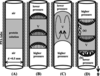Figure 1 Schematic illustrations of the stirring mechanism. (A) Initial state of the protein solution, (B) the Hagen–Poiseuille flow when the droplet is compressed from downward, (C) the axisymmetric rolls when the droplet is moving upward in the tube, and (D) the Hagen–Poiseuille flow in the reverse direction. The stirring is carried out by periodically repeating the processes from (A) to (D). The crystals precipitate (a) on the tube wall or (b) on the air–liquid interface.

The droplet moves a small amount in the tube. At this stage, the frictional force between the droplet and the tube inner wall works as a driving force to induce the flows, and the axisymmetric rolls circulating around the droplet arise, as shown in Fig. 1(C). Three-dimensional computations under these conditions are presented in Appendix A. After the movement of the droplet, the pressure is gradually reduced until the shape of the air–liquid interface becomes inverted, as shown in Fig. 1(D). By periodically repeating the processes from (A) to (D) in Fig. 1, solution stirring is achieved.

In our experiments the crystals precipitated on the tube wall [see Fig. 1D(a)], or on the air–liquid interface [see Fig. 1D(b)]. Since the droplet was held by the friction with the capillary inner wall, the pressure inside the tube was carefully controlled to keep the droplet at a certain position during the course of the experiment. The methods for controlling the pressure and enclosing a nl-order protein solution in a small tube are given in Appendix B.

It should be noted that the protein droplet has to be attached to the inner tube wall directly in order to achieve Hagen–Poiseuille flow. If there is no friction between the droplet and the inner tube wall, then neither Hagen–Poiseuille flow nor deformation of the droplet will be achieved.

### 3. Crystallization

Hen egg-white lysozyme (6X recrystallized, Seikagaku Co. Ltd) was crystallized in a PFA (tetrafluoroethylene perfluoroalkoxy vinyl ether copolymer) tube by a batch method. The inner diameter of the tube was 0.5 mm. The crystallization solution included 25 mg ml−1 lysozyme, 0.1 M sodium acetate and 5% sodium chloride. The temperature was kept at 293 K.

### 4. Viscosity and velocity measurements

The viscosity of a 25 mg ml−1 lysozyme solution at 293 K was measured using a rotating viscometer (RE550R, Toki Sangyo Co. Ltd), which measures the running torque of a round disc that rotates with a constant speed in a solution. Since the torque is governed by the shearing stress of a solution, the viscosity is determined by reference to Newton's viscous law (Rouse, 1946).

The viscosities of 25 mg ml−1 lysozyme solution at 293 K under rotating speeds of 20, 50 and 100 r min−1 were (4.64 ± 0.59) × 10−3, (3.26 ± 0.48) × 10−3 and (2.14 ± 0.18) × 10−3 Pa s, respectively. The decrease in viscosity with increased stirring velocity, as shown in Fig. 2(a), results from thixotropy of a lysozyme solution (Giordano et al., 1981). The rotating speeds of 20–100 r min−1 correspond to 26–131 mm s−1, and these speeds are much larger than those produced by our stirring system. Therefore, the viscosity at 0 r min−1 [(5.86 ± 0.86) × 10−3 Pa s] was determined by extrapolation of the data shown in Fig. 2(b).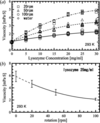Figure 2 The viscosities of the lysozyme solutions. (a) The viscosities measured under various lysozyme concentrations and rotating speeds of the viscometer. Since the lysozyme solution has thixotropy, each viscosity at 20, 50 and 100 r min−1 was approximated with least-squares lines by the quadratic polynomial. (b) The viscosity of 25 mg ml−1 lysozyme solution at 20, 50 and 100 r min−1. These values have 12.66, 14.66 and 8.38% errors, respectively. The viscosity at 0 r min−1 was extrapolated from the least-squares lines. We calculated that the value was (5.86 ± 0.86) × 10−3 Pa s. The error of 15% was estimated from the maximum error of the measurements.

The relationship between the magnitude of stirring and protein crystallization was characterized by the Reynolds number (Re). The Re number, defined as Re = ρvd/μ, is a non-dimensional parameter for characterizing flow, and provides a deeper insight into the stirring mechanism. Here, ρ, v, d and μ are the density (kg m−3), flow velocity (m s−1), representative length (m) and viscosity (Pa s), respectively. The inner diameter of the tube (5.0 × 10−3 m) was defined as the representative length (Rouse, 1946). The velocity at the centre of the air–liquid interface of the droplet was used to calculate the Re number since velocities at the axial centre in the tube became the maximum in Hagen–Poiseuille flow. The density measured by a glass densimeter (5 ml) was 1.04 × 103 kg m−3.

### 5. Results

We performed four different stirring experiments (cases A–D). These results are presented in Figs. 3(A)–3(D), respectively. Fig. 3(E) shows the results of a control experiment (no flow).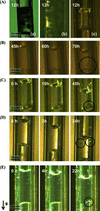Figure 3 The crystallization processes in PFA tubes. The solution volume was 100–200 nl and the stirring conditions were as follows. (A) Continuous stirring (a) v = 5.3 × 10−4, Re = (4.7 ± 0.3) × 10−2, (b) v = 1.6 × 10−5, Re = (1.4 ± 0.1) × 10−3 and (c) v = 2.5 × 10−6 m s−1, Re = (2.2 ± 0.2) × 10−4. (B) Stirring period 4 s and resting period 4 min 56 s. v = 5.0 × 10−6 m s−1, Re = (4.4 ± 0.3) × 10−4. (C) Stirring period 4 s and resting period 14 min 56 s. v and Re under the stirring are the same as in cases (B) and (C). (D) Stirring period 4 min and resting period 11 min. v = 0.4 × 10−6 m s−1, Re = (3.5 ± 0.2) × 10−5. (E) Control experiment. Lysozyme crystals are marked by the black circles.

#### 5.1. Case (A)

Crystallization under continuous vibration of the droplet with flow velocities v = 5.3 × 10−4 and 1.6 × 10−5 m s−1 resulted in only spherulite (needle crystals) after 12 h, as shown in Figs. 3(A)(a) and (b). When the velocity was decreased to v = 2.5 × 10−6 mm s−1, many small crystals appeared after 12 h, as shown in Fig. 3(A)(c). The Re numbers in the experiments shown in Figs. 3(A)(a), (b) and (c) were (4.7 ± 0.3) × 10−2, (1.4 ± 0.1) × 10−3 and (2.2 ± 0.2) × 10−4, respectively.

The changes in the position of the droplet exhibited a certain time delay in response to the changes in pressure of the air phase. Owing to this time delay, continuous vibration (case A) caused the gradual drift of the average position of the droplet during the course of the crystallization experiment. In contrast, in cases (B)–(D), intermittent resting periods were imposed between the processes (C) and (D), and the processes (D) and (B) in Fig. 1. This discrete stirring was achieved by pushing, stopping, withdrawing and re-stopping the microsyringe pump sequentially (see Appendix B). Although the magnitude of the stirring was weakened by the presence of resting periods, the homogenization of concentration was periodically achieved. In our experiment, discrete stirring was more reliable than continuous stirring at keeping the droplet at a certain position, and hence allowed vibration of a nl-order microdroplet for a longer time.

#### 5.2. Case (B)

We moved the droplet for 4 s, and rested for 4 min 56 s. One cycle of the microsyringe pump (push, stop, withdraw and re-stop processes) was 10 min. The maximum flow velocity measured at the air–droplet interface was 5.0 × 10−6 m s−1. The droplet was held at the initial position throughout the experiment, and only the air–liquid interface was regularly vibrated. Amorphous precipitates appeared within 60–70 h at the air–liquid interface. The crystallization process is shown in Fig. 3(B). The Re number under the stirring was calculated as (4.4 ± 0.3) × 10−4.

#### 5.3. Case (C)

We remarked on the importance of the resting period. The droplet was moved for 4 s and rested for 14 min 56 s. One cycle of the pump was 30 min, and the difference between case (B) and case (C) was only the length of the resting period. The Re number under the vibration was the same as that of case (B). The crystallization process is shown in Fig. 3(C). The droplet in the tube vibrated at the initial position throughout the experiment. In this case, one bulk lysozyme crystal appeared within 10 h at the air–liquid interface, and grew to about 0.3 mm. This result clearly demonstrates that the resting period significantly influences protein crystallization.

#### 5.4. Case (D)

In this case, we adopted a flow velocity smaller than that used in cases (B) and (C). The moving period was for 4 min, and the resting period for 11 min. The flow velocity was 0.4 × 10−6 m s−1, and the pump cycle was 30 min. The droplet moved about 0.7 mm downward, keeping the vibration. The crystallization process is shown in Fig. 3(D). Two crystals appeared in 3 h on the inner tube wall, and grew to the same size (0.1 mm). The waiting time of nucleation and the crystal size were the same as those in the control experiment (see Fig. 3E). The Re number was (3.5 ± 0.2) × 10−5.

### 6. Discussion

The results in case (A) [Figs. 3A(a), (b), (c)] show that stirring by continuous vibration was too strong. In contrast, cases (B)–(D) included resting periods after the stirring. In cases (B) and (C), both flow velocities under the vibration were the same, but the resting period in case (C) was three times longer than that in case (B). Low-Reynolds-number flow within a range of Re < 1 is commonly called Stokes flow, and the inertia term in the Navier–Stokes equation [see equation (1) in Appendix A] becomes negligibly smaller than the viscous term and the pressure term (Happel & Brenner, 1973). Therefore, before the experiments, we had expected that the influence of the resting time (continuous stirring or discrete stirring) might be small. However, we obtained only one bulk crystal in case (C), and amorphous precipitates in case (B). These results demonstrate that stirring has a much more significant influence on protein crystallization than we expected. We found that protein crystallization is very sensitive to flows, and the optimum Re number for protein crystallization is in the range of 10−4 < Re < 10−5, which is much smaller than values reported in previous studies.

### 7. Conclusions

We have developed a new stirring method and examined the relationship between stirring and protein crystallization. Our method is able to stir nl-order protein solutions with a well defined flow velocity, and allows us to observe droplet movement and crystallization processes. The flow pattern under stirring was easily analysed by observation of the solution. Using this method, we evaluated the Re number under stirring and carried out crystallization experiments. We found that protein crystallization is significantly influenced by a flow with extremely small Re number. We believe that our stirring method will open up new possibilities for protein crystallization using sample solutions on a nl scale and for detailed studies on the effect of flow.

### Three-dimensional computations of flow in the droplet moving in the tube

The non-dimensional Navier–Stokes equation and the equation of continuity are given by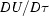=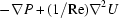(equation 1) and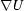= 0 (equation 2), respectively, where U, τ, P and Re denote the non-dimensionalized velocity vector, time, pressure and the Reynolds number, respectively. The representative length d is a diameter of a tube. Three-dimensional computations were carried out to solve equations (1) and (2) explicitly. When the droplet moves upward in the tube, the frictional force directs downward. Here, the boundary conditions at the side wall were given as Re = 1.0 × 10−4 and 1.0 × 10−1, directing downward. On the other hand, a frictionless condition is considered on the top and bottom interfaces. The programming language is Fortran (Compaq Visual Fortran Professional Edition, Compaq Co.), and the results are shown in Fig. 4. The computational grid of the cylindrical coordinate system is shown in Fig. 4(a). The aspect ratio (= diameter/height) is 1.0. Figs. 4(b) and 4(c) show the streamlines of Re = 1.0 × 10−4 and 1.0 × 10−1 cut on the X = 0 and Y = 0 planes. The streamlines are quite similar, and the axisymmetric rolls occur in the droplet. Both computations (Re = 1.0 × 10−4 and 1.0 × 10−1) converged within τ = 1.0 × 10−5 and 0.01, respectively. These results indicate that the flows take place immediately after the droplet moves. The velocity distributions of the Re = 1.0 × 10−4 flows cut in the X = 0 plane are shown in Fig. 4(d).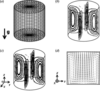Figure 4 Three-dimensional computations of flow in a moving droplet enclosed in a cylindrical tube. (a) Computational grid of the cylindrical coordinate system. Mesh number (R, θ, Z) = (10, 41, 20), (b) the streamlines of Re = 1.0 × 10−4 flows cut on the X = 0 and Y = 0 planes, (c) the streamlines of Re = 1.0 × 10−1 flows cut on the X = 0 and Y = 0 planes, and (d) the velocity distributions of the rolls cut in the X = 0 plane. The convergence condition for the equation of continuity is less than 10−4.

### Experimental equipment

Fig. 5is a schematic illustration of the equipment. A protein droplet (100–200 nl) vibrates in a vertically standing PFA tube, as shown in Fig. 3. The vibration was produced by a periodical pressure difference, which was guided by a piston motion precisely controlled by a microsyringe pump (PHD200-P, Harvard Co. Ltd).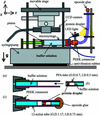Figure 5 A schematic illustration of our stirring system. (a), (b) and (c) show the procedure to set up the 100–200 nl protein droplet in the tube.

In order to place the protein droplet in the tube, first the microsyringes (1705 TLI-50 µl SYR, Hamilton Co. Ltd) and a 12-nylon tube (inner diameter 0.73 mm, outer diameter 1.17 mm) were filled with a buffer solution (blue region in Fig. 5a). By using a small micropipette, the buffer solution and the 100–200 nl protein solution were enclosed in a PFA tube (inner diameter 0.5 mm, outer diameter 0.7 mm), as shown in Fig. 5(b). Next, the PFA tube was placed inside the 12-nylon tube, as shown in Fig. 5(c). Finally, a two-component epoxide adhesive (quick-drying araldite, Huntsman Advanced Materials Co. Ltd) was used to plug the gap between the tubes.

This procedure is convenient for quickly enclosing a nl-order protein droplet in a small-diameter tube, and for decreasing air volume to about 5 µl. Since the air volume is so small, contamination and drying of the droplet are prevented.

The crystallization process was recorded using a CCD camera (CS9001, Toshiba Teli Co. Ltd) that was attached over the microsyringe pump. The digital images taken by the camera were preserved on a PC (using the software Image Pro-Plus, version 5.1, Media Cybernetics Co. Ltd). The focus of the CCD camera was adjusted using a movable stage. An antivibration rubber was used to absorb external vibration. An LED light source was used to avoid rising temperatures. The whole experimental setup was placed inside an incubator to control the temperature at 293 ± 0.1 K.

### Acknowledgements

This research was supported by CREST of the Japan Science and Technology Corporation (JST). The viscosity was measured at the Industrial Technology Center of Wakayama Prefecture.

### References

This is an open-access article distributed under the terms of the Creative Commons Attribution (CC-BY) Licence, which permits unrestricted use, distribution, and reproduction in any medium, provided the original authors and source are cited.JOURNAL OFSYNCHROTRONRADIATION
ISSN: 1600-5775
Volume 15| Part 3| May 2008| Pages 269-272